### Multivariable Calculus

Understanding graphs and surfaces requires us to delve a little deeper into the place where they live: $\mathbb{R}^3.$

This quiz covers the essentials of three-dimensional coordinate systems. We'll need some right triangle trigonometry in order to construct the polar, cylindrical, and spherical coordinate systems.

We'll start with the 3D Cartesian system, which extends the familiar 2D $xy$-coordinate system into the full three dimensions of our day-to-day experience by adding a new axis. (Check out the animation below!)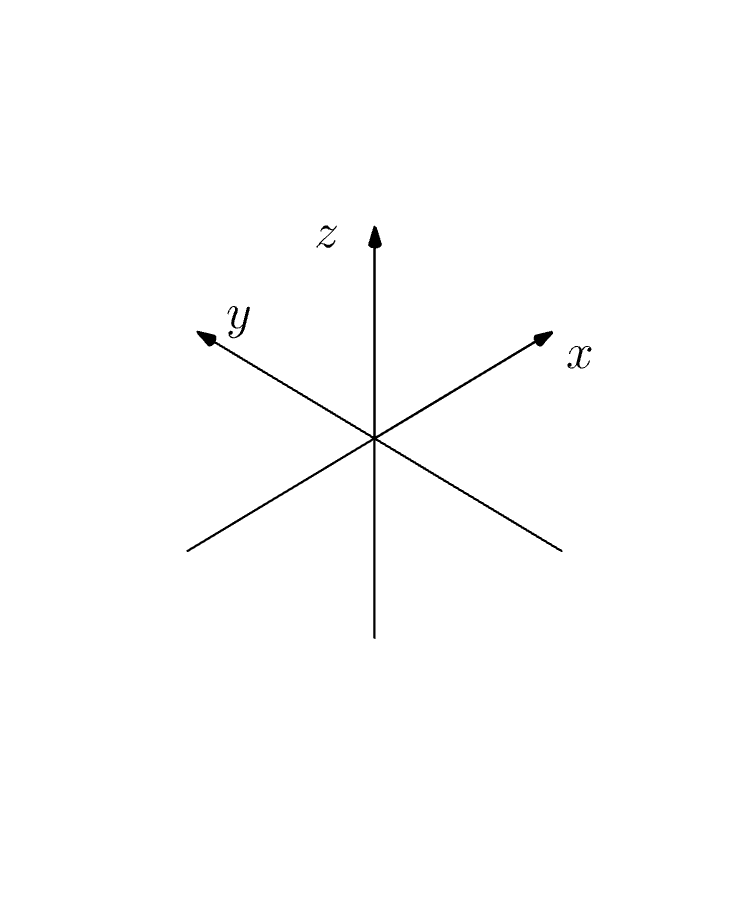# Coordinates in 3D

The new axis is labeled $z,$ and we have three coordinates $( x,y,z)$ for every point in space $\mathbb{R}^3.$

To get to the point $(1, 2 , 2)$ pictured below (red dot), we start at the origin where all axes meet, move 1 unit in the positive $x$ direction, 2 units in the positive $y$ direction, and then 2 units up in the positive $z$ direction.

What are the coordinates of the green triangle?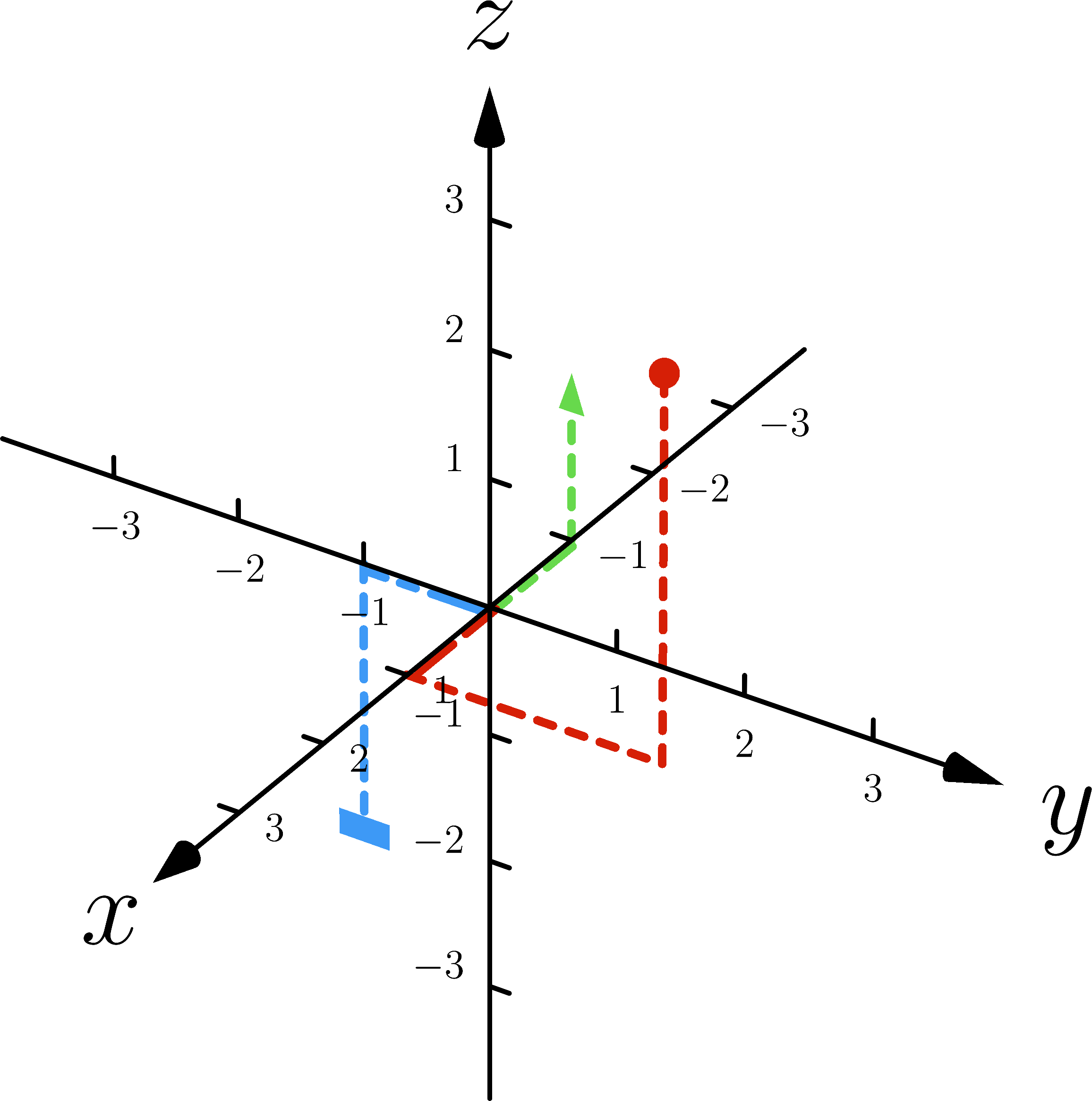Note that you are able to toggle and zoom into the diagram above.

# Coordinates in 3D

Now, what are the coordinates of the blue rectangle?# Coordinates in 3D

Not all creatures prefer Cartesian coordinates.

Bees do a waggle-dance to communicate distance and direction information, in effect using a coordinate system based on circles instead of perpendicular lines.

If we're to be at least as clever as bees, we should develop alternative coordinate systems, too!

Cylindrical and spherical coordinate systems for $\mathbb{R}^3$ are just such coordinate systems, and both are built up from polar coordinates $(r,\theta)$ in $\mathbb{R}^2,$ the system of choice for talkative bees.

The rest of the quiz will develop all three coordinate systems from scratch.

# Coordinates in 3DWe arrive at the planar point $P =(x,y)$ by following a ray starting at the origin and making an angle of $\theta$ with respect to the positive $x$-axis for a distance of $r.$

Trigonometry then tells us that $x = r \cos(\theta ).$ What option best represents the relationship between $y$ and the polar coordinates $(r,\theta) ?$

# Coordinates in 3D

Polar coordinates and Cartesian coordinates are related through $x = r \cos(\theta), \quad y = r \sin(\theta) .$ Suppose we're given the Cartesian coordinates for $P$ but want to know the polar form. Since $\sin^2(\theta)+ \cos^2(\theta) =1 ,$ \begin{aligned} \sqrt{x^2+y^2} &= \sqrt{ \big[ r \cos(\theta) \big]^2 + \big[r \sin(\theta)\big]^2} \\ & = \sqrt{ r^2 \big[ \cos^2(\theta)+ \sin^2(\theta)\big]} \\ &= \sqrt{r^2 } \\ &= r. \end{aligned} What formula lets us calculate $\theta$ given the Cartesian coordinates $(x,y)?$

# Coordinates in 3D

For 3D problems, we can build a hybrid of the 2D polar system and Cartesian coordinates called polar cylindrical (or just cylindrical) coordinates.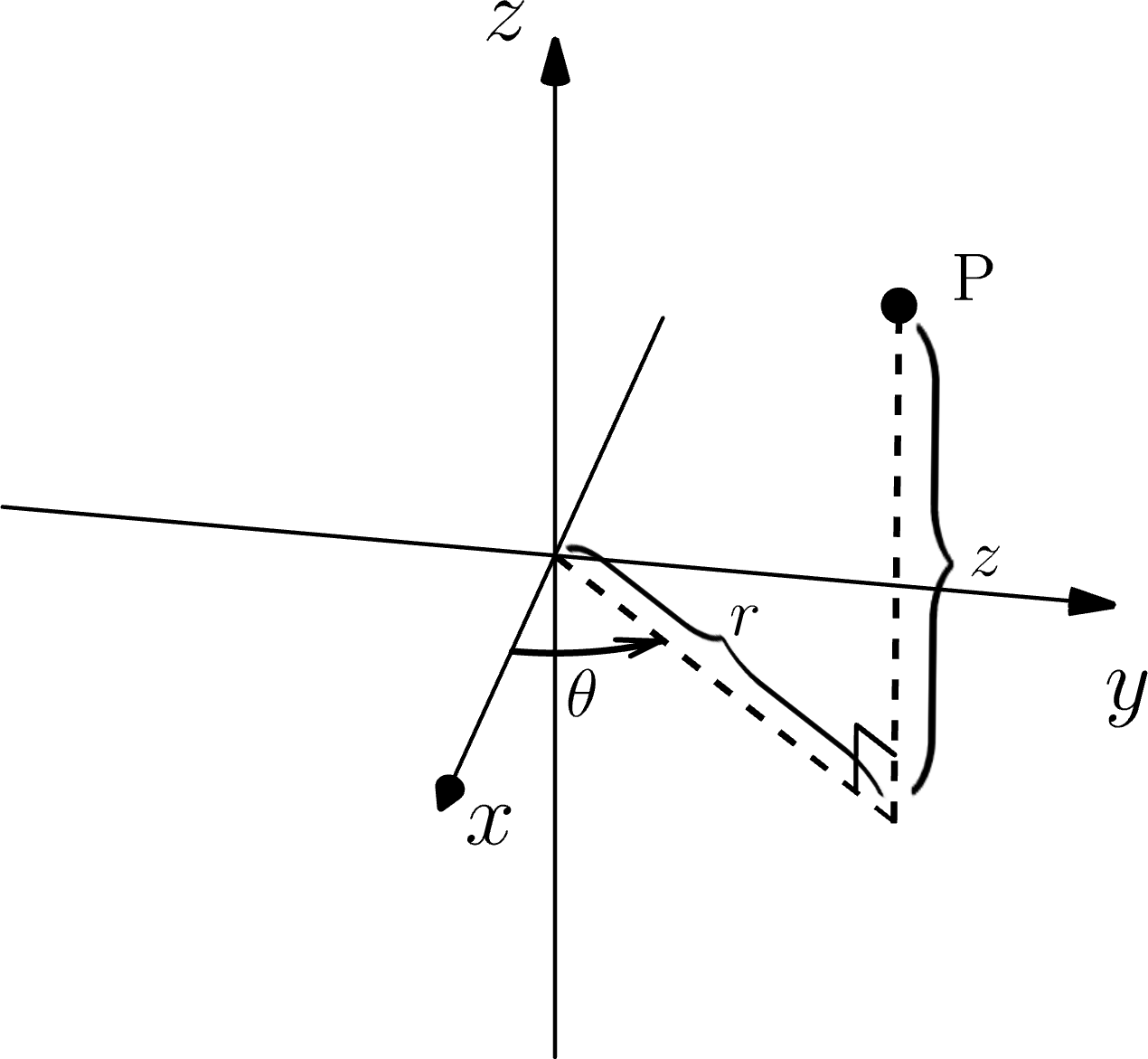Suppose $P = (x,y,z) \in \mathbb{R}^3.$ The first two numbers represent a point in the plane, which we can describe using $( r, \theta).$ The cylindrical coordinates of $P$ are then $(r,\theta,z).$

# Coordinates in 3D

Compute the cylindrical coordinates of $(-2,-2, 5).$ The interactive below may help.

Adjust the controls until the point sits at the top of the vertical line....

# Coordinates in 3D

Cylindrical coordinates are ideally suited for problems in $\mathbb{R}^3$ symmetric about the $z$-axis, like describing $C_{R},$ the cylinder of radius $R$ about the $z$-axis, which is made up of all points of distance $R$ from this line.

Of the equations presented, which one best describes $C_{R}$ in cylindrical coordinates?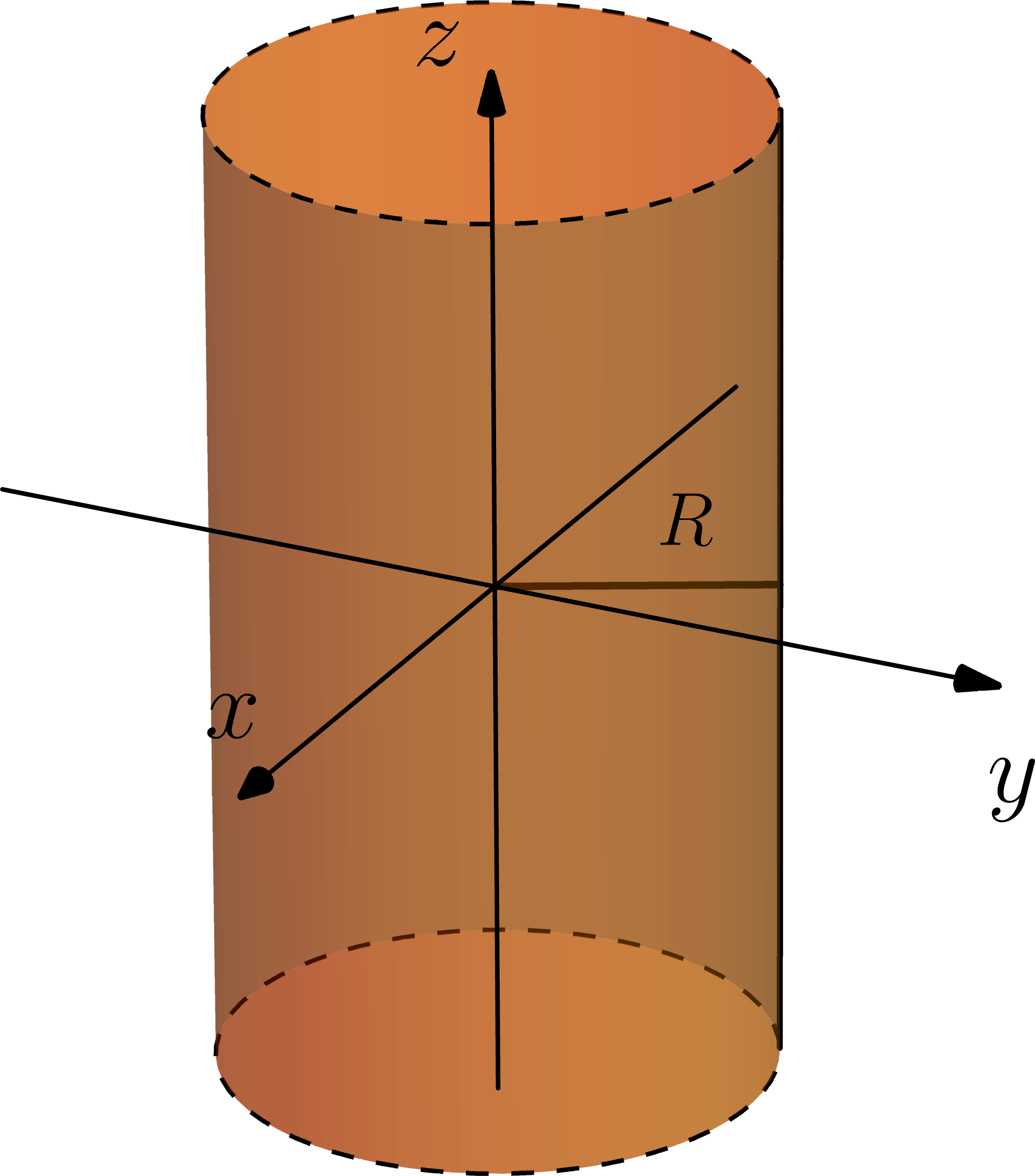Note: We use dashed lines to indicate portions of the picture that continue out to infinity. The cylinder above continues parallel to the $z$-axis in both the positive and negative directions.

# Coordinates in 3D

When a problem has complete symmetry around the origin, spherical coordinates are usually better than cylindrical coordinates.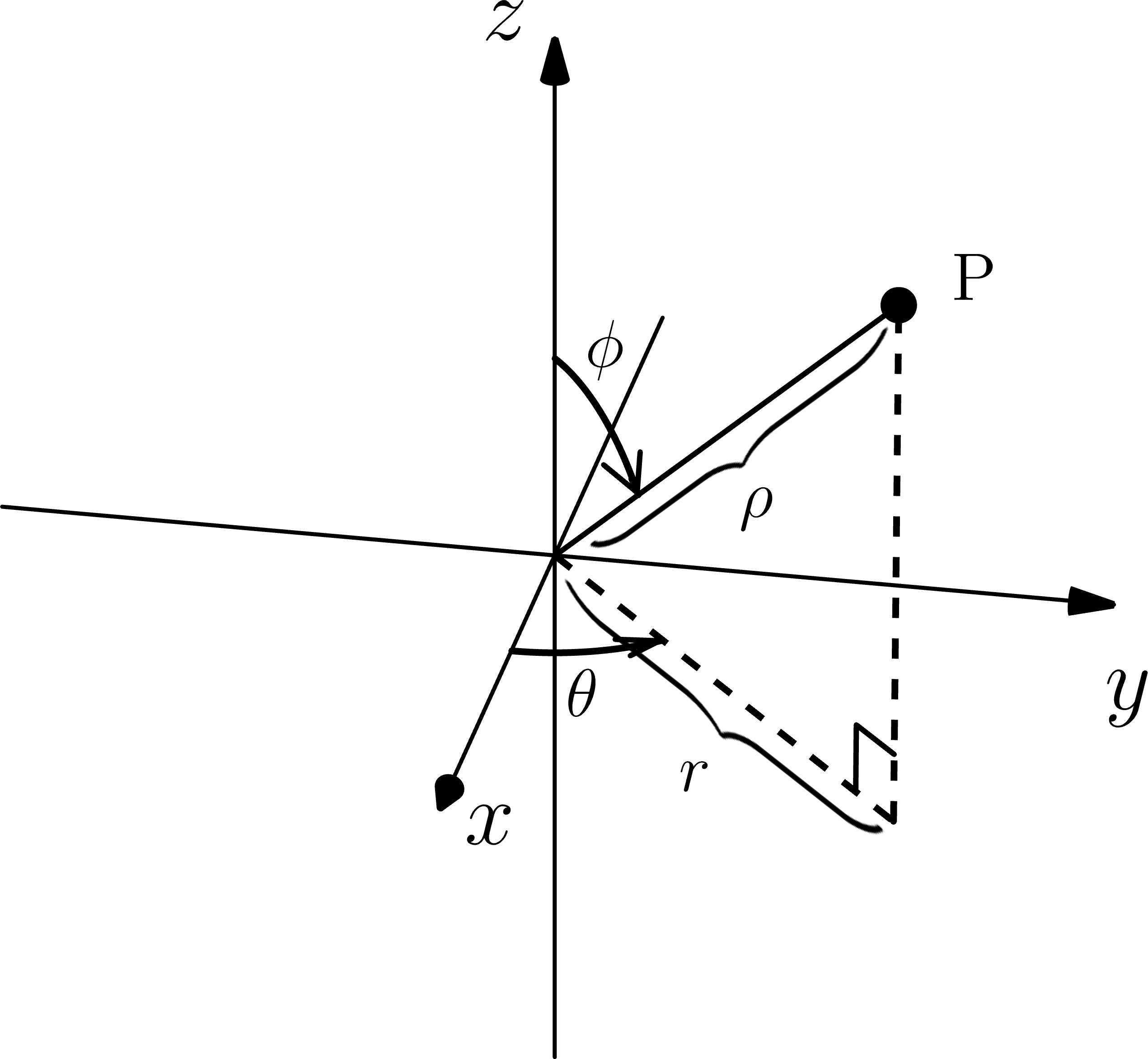Suppose $P = (x,y,z) \in \mathbb{R}^3.$ We know $x = r \cos(\theta),\quad y = r \sin(\theta) ,$ where $r$ is the distance between the point $( 0,0)$ and $(x,y)$ in the plane.

$\theta$ is taken to be one of the new spherical coordinates; the other two are $\rho$ $\big($the distance between $P$ and $(0,0,0)\big)$ and $\phi,$ the angle between the positive $z$-axis and the ray joining $P$ with the origin.

Use the diagram to relate $r$ and $\rho.$

Hint: It's a geometric fact that the angle made by the solid and dashed lines meeting at $P$ form an angle of size $\phi$ there.

# Coordinates in 3D

Since $r = \rho \sin(\phi),$ $x = \rho \sin(\phi) \cos(\theta), \quad y = \rho \sin(\phi) \sin(\theta).$ We need to find a formula for $z$ to complete the relationship between Cartesian and spherical coordinates.Using the picture above, what is $z$ expressed in terms of $( \rho, \theta, \phi) ?$

Hint: Remember from the last problem that the angle made by the lines meeting at $P$ has size $\phi,$ too.

# Coordinates in 3D

Finally, let's understand why the coordinates $( \rho, \theta, \phi)$ given by $x = \rho \sin(\phi) \cos(\theta),\quad y = \rho \sin(\phi) \sin(\theta),\quad z = \rho \cos( \phi)$ are called spherical.

Of the options presented, which one correctly describes the sphere $S_{R}$ of radius $R$ centered at the origin in spherical coordinates?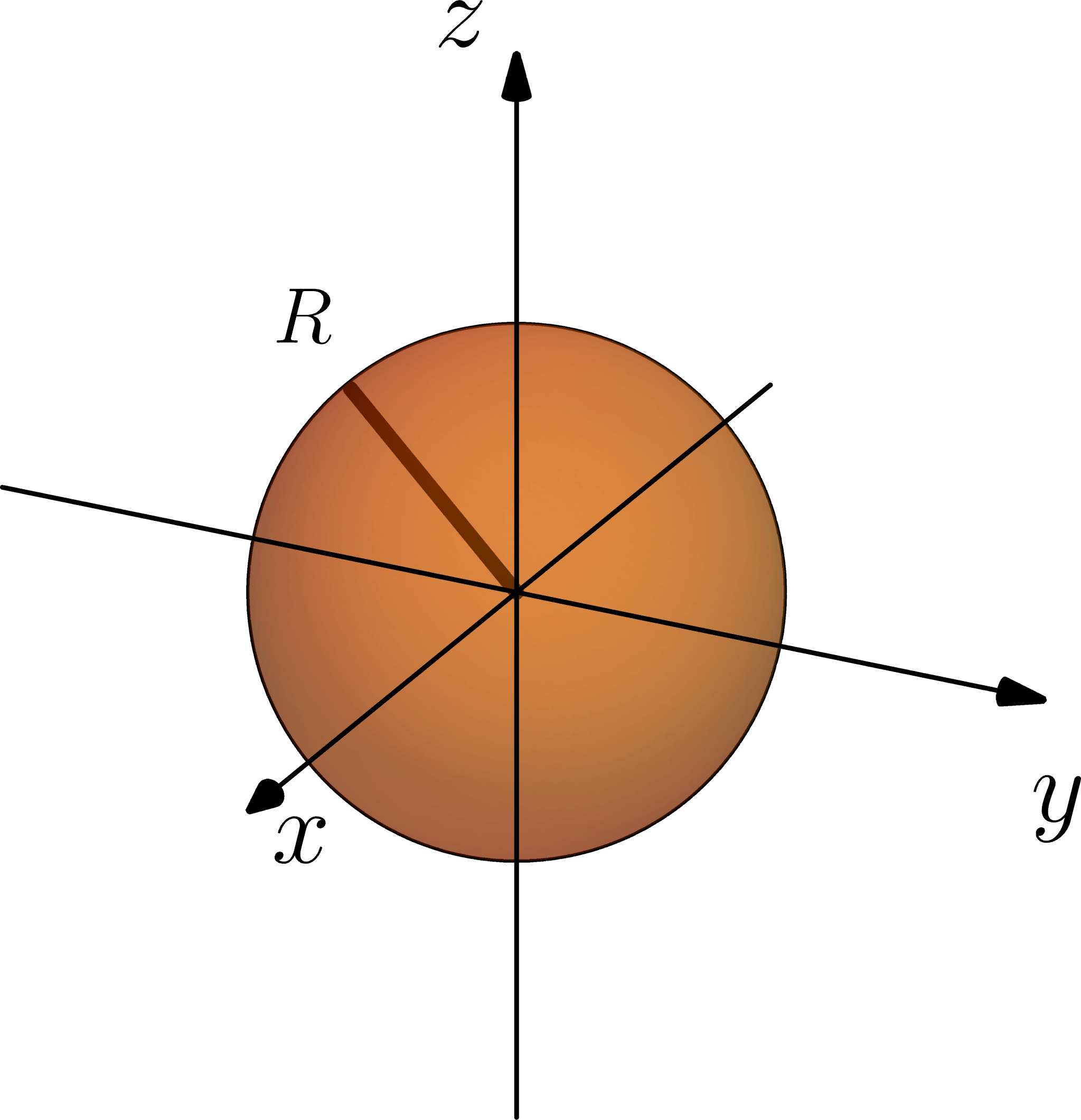# Coordinates in 3D

Visualizing mathematical objects like surfaces in $\mathbb{R}^3$ can be very helpful in solving many multivariable calculus problems.

We saw one example of this already when we visualized the graph of the depth function at the end of the optimization quiz. At a glance we were able to see where the minimum and maximum values of the depth function occur.

The Cartesian, spherical, and cylindrical systems provide the means for visualizing a large variety of useful objects in multivariable calculus.

The final quiz of this intro chapter shows one particular and very important example.

There, we'll use the 3D coordinate system to understand what place integrals have in the world of multivariable calculus.

# Coordinates in 3D

×